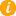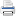Math K-Plus

# Practice 3rd Grade Division Calculator

TestQuizQuiz Results• Option 2. Solve a calculator generated math problem. First press the NEW PROBLEM button. Next enter your answer, in to the calculator. Lastly, press the ADD button and then compare your answer with the calculator's.

Field Name Divide Values
Dividend: You can enter only 2 digit
Divisor Definition: You can enter only 2 digit
÷
You can enter up to 6 digitsAfter learning third grade division, you can move to the next step in division math: Fourth Grade Division Calculator.

## Problem: Divide Positive Integer Numbers.STEP 0. +15√+63  15√ 63 STEP 1.     4  15√63    -60     3 ANSWER: 4 3/15 4 Remainder 3

The calculator above has a number of valuable features: